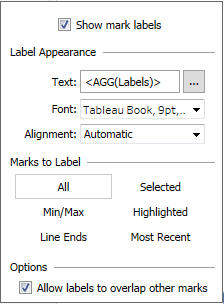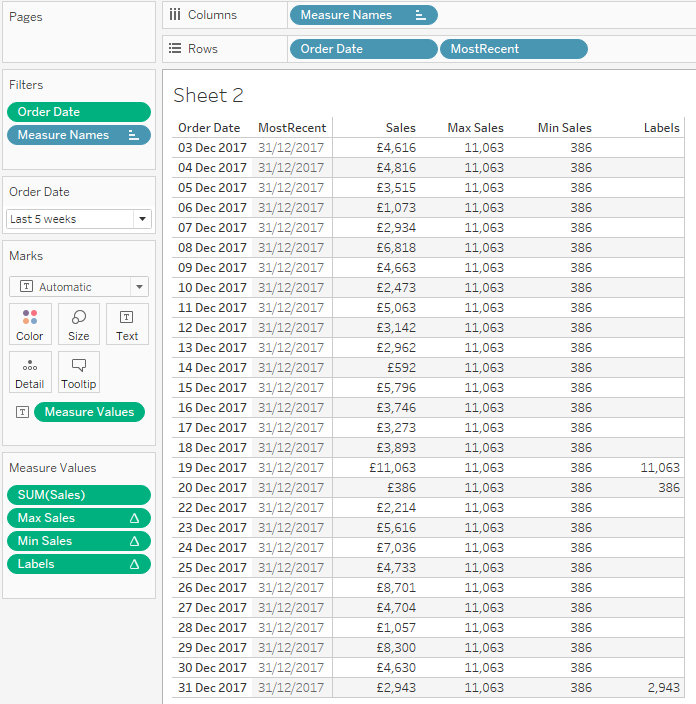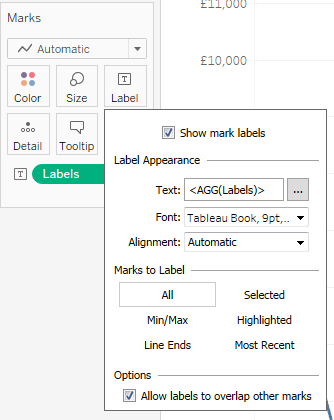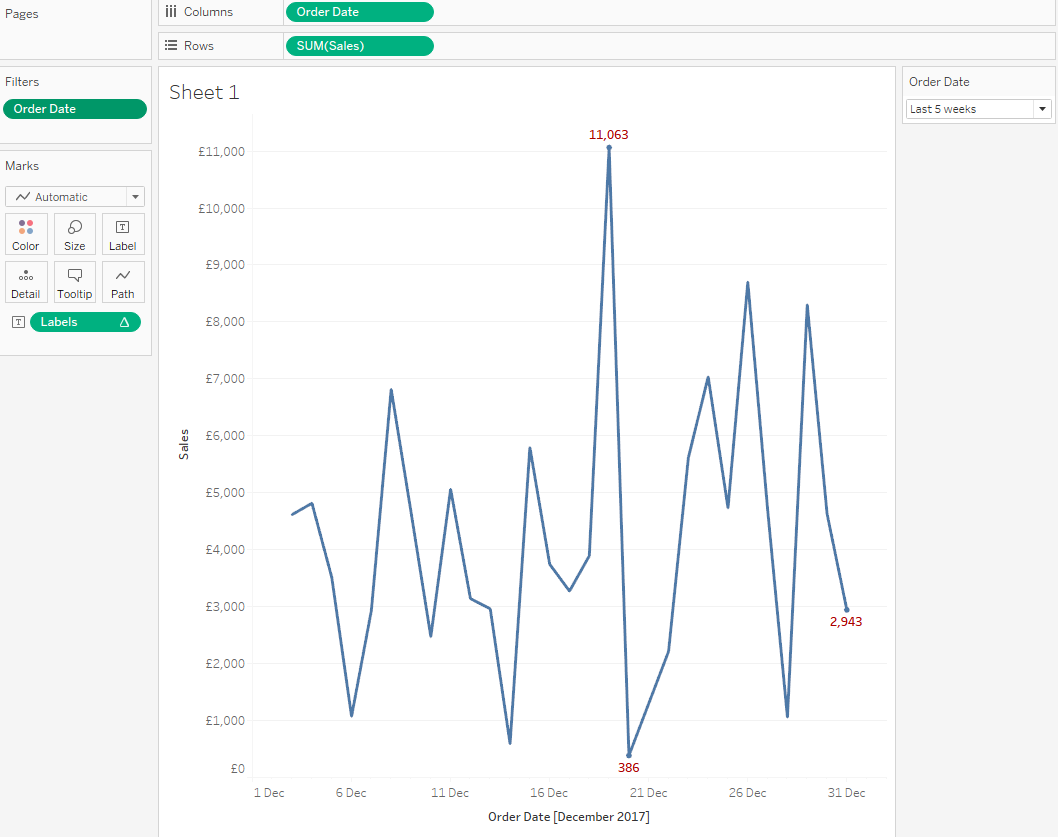The standard label options for line charts in tableau give a lot of flexibility. But what if you want to do something non-standard, something that’s not one of the default options?

Standard options for labels are quite comprehensive, including:

• All points
• Min and / or Max points
• Start and End points of the lines
• The Most Recent pointPerhaps within your Tableau line chart you want to show labels for the Min, Max AND the Most Recent values. This post will show you how to do this.

As with most things in Tableau, this can be achieved with calculations.

Line charts generally show a measure over time. Therefore we want a calculation that returns Null for all dates except those with the maximum, minimum and latest values.

Breaking the calculation into small chunks is recommended. We need to be able to isolate the following:

1. The Maximum value
2. The Minimum value
3. The Most Recent date

For this example I’ll use the sample Superstore data source, I want to show a trend of sales over time, with labels showing the minimum, maximum and latest values.

Firstly the Maximum value. This can be done using LOD calcs or table calculations. I’m using a table calculation as I want flexibility, with the maximum value changing dependent on the date filter. (LODs would mean having to getting more complicated with context filters, which I prefer to avoid.)

Max Sales: WINDOW_MAX(SUM([Sales]))

Next the Minimum value, almost the same as above:

Min Sales: WINDOW_MIN(SUM([Sales]))

The Most Recent date. This time I will use a LOD. This could also be changed to a table calculation should I want flexibility to move the latest date. For this example a LOD will suffice:

MostRecent: {MAX([Order Date])}

Now I can bring these together creating the Labels calculated field:

Labels:

IF [Max Sales] = SUM([Sales]) //Max Sales
OR [Min Sales] = SUM([Sales]) //Min Sales
OR ATTR([Order Date]) = MAX([MostRecent]) //Latest date
THEN SUM([Sales])
END

This returns the sales amount for the minimum and maximum dates and also return the sales for the most recent date. Ensure the table calculations are set to compute using the Order Date.

To test it works set it up in a simple table.Migrating this to a line chart is straightforward, simply put the field [Labels] on the Label shelf and make sure the Marks to Label is set to All.The final worksheet looks like this, including some minor formatting of the label colour: